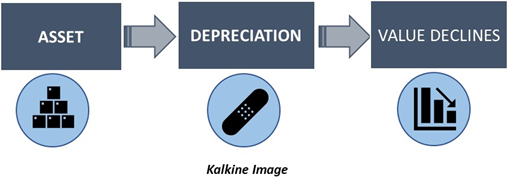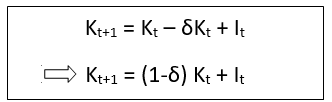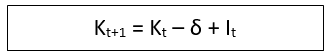# Economic Depreciation

• Updated on

## What is Economic Depreciation?

Economic depreciation refers to a decrease in the value of an item or an asset over time. The decline in value reduces the price of the asset in the market. Assets generally get depreciated because of the wear and tear.

Economic depreciation is different from the depreciation used in accounting. In accounting, depreciation is the method of spreading the cost of an asset over time. This is done to calculate and record the value of an asset in the balance sheet.

Economic depreciation becomes more visible as time passes or as external factors change. This leads to a reduction in the market value of the asset. In such cases, if the assets are resold, they fetch a lower amount compared to what was paid to acquire them.## How is economic depreciation recorded in the system of national accounts?

Economically, depreciation is recorded as the reduction in income owing to the loss in value of capital. Depreciation is also known as the “Consumption of Fixed Capital” for this reason.

While moving from “gross” measurements to “net” measurements, depreciation is reduced from the gross value of the variable. For instance, Net GDP can be calculated by subtracting depreciation from gross GDP.

There are two significant elements that lead to the decline in value of an asset:

• First, the value may change because of changes in the prices of the asset class itself due to time difference. For instance, measuring the value of a new asset in the current year and then again after a year.
• Secondly, the value may also change because of the ageing of the asset. For instance, comparing the price of a new item bought this year to the price of a one-year old item.

Together, these two factors affect how prices of a good change with time. However, in a strict sense of the term, depreciation may only account for the second factor.

## Why do assets depreciate?

Assets may depreciate because of the normal process of ageing or because of certain external factors. These include:

• Normal Wear and Tear: Goods that last a long term are bound to get affected because of constant usage. For instance, the machine equipment in a firm might function smoothly for the initial years. However, it starts to malfunction and becomes slower at later stages. This is depreciation caused by wear and tear of the machine and is reflected in its market value. Therefore, if the company were to resell the machine after using it for a few years, then the resale value would be lower as the machine is not in the same condition, physically, as it was before.
• Obsolescence: Parts of machinery and equipment might become obsolete with time. Similarly, old machines might get replaced with equipment that has newer technology in it which makes it more efficient. Therefore, the old or obsolete equipment would be more susceptible to depreciation as repairs may not be possible on them.
• Normal accidental Damage: This refers to the accidents encountered in the workplace that can cause an asset to depreciate. These accidents are the kind of mishaps that are commonly encountered in the production process. This ultimately leads to these assets being scrapped prematurely. Transport equipment might be more vulnerable to these kinds of damages.
• Time to expiry: Items that have an expiry date associated with them or those that are perishable are susceptible to loss in value over time. As the expiration date comes closer, the value of the item decreases. Similarly, intangible assets like intellectual property rights, patents, etc., also have expiry dates attached to them. Therefore, as these rights expire, the item or commodity against which the right was granted loses its market value.

## How is economic depreciation calculated?

There are two methods of measuring depreciation. These are:

• Through the value of goods produced with the asset: The depreciation of machinery or equipment can be measured by the value of the output it produces. For instance, if a machine can manufacture 40 units of a good in a day, then it is more valuable than if it were to make 20 units in a day. If the good is priced at US\$5, then the initial value of goods produced by the machine is US\$200, but after depreciation, the value becomes US\$100. Therefore, the depreciation, in this case amounts to US\$100.
• Through the resale value: Depreciation can also be calculated through the decline in the resale value. The difference between the initial price of the asset and the resale value can be termed as depreciation.

## How does depreciation affect the capital stock in an economy?

In macroeconomics, economic depreciation is subtracted from the current capital stock to calculate the future capital stock. Depreciation can be subtracted as a proportion of the existing stock or as a constant value from the existing stock.

When depreciation is reduced as a proportion of capital stock, then the capital stock in the next period is calculated as follows:Where Kt+1 refers to the capital stock in the next period,

Kt refers to the capital stock in the current period,

δ refers to depreciation, and,

It refers to the level of investment in the economy.

When depreciation is taken as a constant value that is not proportionate to the existing current stock, then the next period capital stock is calculated as follows:The meaning of the variables remains unchanged.

##### Top ASX Listed Companies

Definition:
We use cookies to ensure that we give you the best experience on our website. If you continue to use this site we will assume that you are happy with it. OK